# Outlier

(in statistics)

Any observation in a set of data that is inconsistent with the remainder of the observations in that data set. The outlier is inconsistent in the sense that it is not indicative of possible future behaviour of data sets coming from the same source. Outliers sometimes go by the name of contaminants, spurious or rogue observations, or discordant values.

Valid inferences concerning a particular data set can only be made once one first determines which observations, if any, are potential outliers, and how these observations should be treated in the subsequent analysis. These considerations lead to three main issues associated with outliers: outlier testing, efficient or accommodative estimation, and robust estimation.

Before these issues can be discussed, however, one needs to formulate a mathematical model that describes outliers. One of the most commonly used models, which was originally introduced in its most general form in [a4], is called the slippage model. In this model it is assumed that, out ofobservations,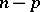arise from some probability distribution with probability density function(cf. also Density of a probability distribution), while the remaining observations (the outliers) come from some modified form of the original distribution, usually denoted by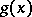. For example,could be the normalprobability density function (cf. Normal distribution), whilecould be. Another model, called the mixture model, assumes that, for each observation, there is a certain probabilitythat the observation comes from the probability density functionand a probability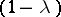that it comes from the probability density function. There are many other outlier models; they can all be found in [a1] or [a6], which are both general reference books on outliers.

The first step in effectively dealing with outliers is to perform a statistical test to determine which observations are potential outliers. In this regard, many test statistics are available for a variety of distributions, many of which have been derived using the maximum-likelihood ratio principle with one of the above-mentioned outlier models as the alternative model. For example, the test statistic(where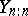is the largest observation,is the average of the observations, andis the sample variance) is the maximum-likelihood ratio test (cf. also Likelihood-ratio test) for the normal model when the alternative model is the slippage outlier model given in the example above with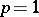and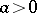. Unusually large values of(given in [a1]) will lead to declaring the largest observation to be an outlier.

Once one has determined that there are potential outliers in the sample, there are two ways to proceed. The first way involves the use of robust methods, that is, methods of analysis that will be minimally affected by the presence of outliers. For example, to estimate the population meanbased on a sample of observations, one might use a trimmed mean,for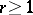, whereare the ordered observations, rather than the full sample mean (which is the trimmed mean with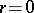). The trimmed mean excludes entirely thelargest andsmallest observations (which are most likely to be the outliers under the slippage model) from the analysis, and can therefore be expected to perform better than the full sample mean when outliers are indeed present. The performance of an estimator can be assessed based on its bias and mean-square error (cf. also Biased estimator; Error). Such comparisons can be found in [a4] and [a5] for the single outlier () normal model. For example, using the slippage model described above when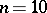, the full sample mean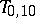has mean-square error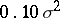when no outliers are present and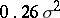when there is a single outlier in the sample with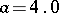. On the other hand, the mean-square error of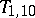iswhen no outliers are present, and only increases to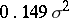when there is a single outlier with. Thus, the robust estimatorprovides protection (considerably lower mean-square error) against the presence of outliers, for a premium (slightly higher mean-square error when there are no outliers).

Recent advances in this area, which deal with the multiple outlier situation (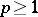) for several different parametric models, can be found in [a2] and [a3].

The second method for dealing with outliers, known as efficient estimation, involves looking for a specific estimator that is optimal for the given data set. In this method, the estimator to be used will vary for different data sets, depending on the size of the sample, the number of potential outliers and how pronounced the outliers are. In fact, when outliers are present, the trimmed mean is not always the best estimator. The reason for this is the fact that the trimmed mean entirely excludes observations that may still contain some useful information about the parameter. A less drastic alternative to the trimmed mean involves the use of an estimator that includes the possible outliers, but gives them less weight. One such estimator is the linearly weighted mean,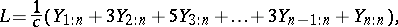which can be used as a robust estimator of the meanfor the normal model. An example of efficient estimation would be to choose between the trimmed mean and linearly weighted mean, depending on which estimator performs the best (lowest mean-square error) for the given data set. Tables to facilitate such a decision for the exponential model,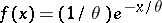, can be found in [a2]. There it is shown that when the outliers are not very pronounced, a weighted type of estimator ofis actually more efficient than a trimmed mean, while the reverse is true when the outliers are far removed from the remainder of the data.